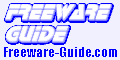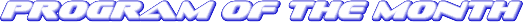(June 2007)

SpeQ Mathematics

(small, extensive mathematics program)

Version: 3.0
OS: Windows 95, 98, ME, 2000, NT, XP or Vista
Release: June 25, 2007Introduction

SpeQ is a small, extensive mathematics program with a simple, intuitive interface. All calculations are entered in a sheet. In there you can freely add, edit and execute all calculations. You can define variables and functions, and plot graphs of your functions. You can save your calculations for later re-use.

Objective

SpeQ is written to be an advanced but very easy to use math program. It is small and fast and runs on every Windows computer, also from an USB-key or floppy disk. SpeQ is usefull for simple, brief calculations as well as working out sheets with extensive variable definitions, functions and complex calculations.

SpeQ is usable for engineers, students and pupils or simply as replacement of the Windows Calculator.

Features

SpeQ has the following features.
• Expression calculations with arithmetic, conditional, logical and bitwise operators
• Around 100 built-in mathematical, physical and conditional constants
• Around 60 built-in functions for Analysis, Arithmetic, Complex numbers, Hyperbolic, Integers, Probability, Numeral systems, Statistics, Trigonometry
• Define your own variables and functions for use in calculations
• Plot fully adaptable graphs, trace the plotted functions, save your graphs
• Decimal, binary, hexadecimal and octal numeral systems
• Calculations with lists
• Complex number calculations
• Colored workarea
• Save and load sheets
• Orderly overview of all available functions and constants in the Functionstree
• A list with all defined variables and functions in the Memorylist
• Context-sensitive help and a detailed users guide
• Enter mathematical expressions in a sheet
• Operators:
• Arithmetic: + - * / % ^
• Conditional: =, <>, <, >, <=, >=
• Logical: And, Or, Xor, Not
• Bitwise: &, |, ||
• Around 100 built-in constants on the area of
• Mathematics: General, Number Theory, Chaos Theory, Combinatorics, Analysis
• Physics: Universal, Electromagnetic, Atomic and Nuclear, Physico-Chemical
• Conditions
• Colors
• Functions:
• Analysis: fnInt, fnDiff
• Arithmetic: Abs, Exp, Ln, Log, Log10, Sqrt, Sign
• Complex numbers: Re, Im, Abs, Arg, Conj
• Hyperbolic: Cosh, Sinh, Tanh, ACosh, ASinh, ATanh, Sech, Csch, Coth
• Integers: Round, Floor, Ceil, Fix
• Probability: Factorial, nCr, nPr, !, Rand, RandInt
• Statistics: Avg, Min, Mean, Max, Prod, Sum, Std, Sort, Var
• Representation: Bin, Dec, Fraction, Hex, Oct
• Trigonometry: Cos, Sin, Tan, ACos, ASin, ATan, Atan2, Sec, Csc, Cot
• Plot graphs
• User-friendly trace, move and zoom functionality
• Analyze intersections, minima and maxima
• Adjust all figure properties in the Propertywindow or in the Workarea
• Available functions: Plot, PlotLogX, PlotLogY, PlotLogLog, RGB, Close, CloseAll
• System functions and variables: Angles, Bytes, Decimals, SciNotation, Representation, Clear, ClearAll
• Define your own variables for use in calculations
• Define your own functions, using one or more variables
• Decimal, binary, hexadecimal and octal numeral systems
• Calculations with lists
• Complex number calculations
• Colored workarea
• Save and load sheets
• Orderly overview of all available functions and constants in the Functionstree
• A list with all defined variables and functions in the Memorylist
• Context-sensitive help and a detailed users guide